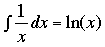SEARCH HOMEMath Central Quandaries & QueriesQuestion from Terry, a student: by separating variables solve the initial value problem (x+1)y' + y = 0 y(0) = 1 Ive done this so far )dy/-y = )dx/x+1 + C ) is integral signTerry,

The completion of this problem requires first that you useand also that you use the properties of logs

log(a × b) = log(a) + log(b), log(a/b) = log(a) - log(b) and log(ab) = b × log(a).

Finally the constant of integration C in your expression is just that, a constant and you can write it any way you wish. In this problem it is helpful to write C = ln(K) for some other constant K.

Try these suggestions and see if you can complete the problem. If you need further assistance tell us what you have done and we will try to help.

HarleyMath Central is supported by the University of Regina and The Pacific Institute for the Mathematical Sciences.